# Maximum Degree of Parallelism in C#

## How to use Maximum Degree of Parallelism in C# with Examples

In this article, I am going to discuss How to use the Maximum Degree of Parallelism in C# with Examples. Please read our previous article, where we discussed Parallel Invoke Method in C# with Examples.

##### How to use Maximum Degree of Parallelism in C#?

Until now, when we use parallelism, we are allowing as many threads as possible from our computer to be used to solve the task that we have. However, this is not necessarily something we are going to want. Sometimes we would want to limit the resources used for a task in order to be able to handle other tasks that we may have pending.

We can configure this by defining the maximum degree of parallelism. With a maximum degree of parallelism, we can indicate how many simultaneous threads we will have working on the code that we want to execute in parallel. For this, we are provided with ParallelOptions Class in C#.

##### ParallelOptions Class in C#

The ParallelOptions class is one of the most useful classes when working with multithreading. This class provides options to limit the number of concurrently executing threads to execute our parallel code as well as provide options to cancel the parallel execution. In this article, we will see how to limit the number of concurrently executing threads and in the next article, I will show you how to cancel the parallel execution with examples.

##### Example without using ParallelOption Class in C#:

In the below example, we are not using the maximum degree of parallelism and hence there is no limit on the number of threads to execute the code parallelly.

```using System;

namespace ParallelProgrammingDemo
{
class Program
{
static void Main(string[] args)
{
Parallel.For(1, 11, i =>
{
});
}
}
}
```
###### Output: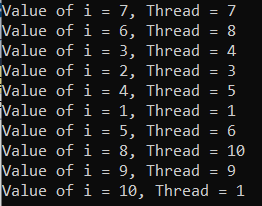As you can see in the above output there is no limit on the number of threads to execute the code. Now, suppose we want a maximum of three threads to execute our code. Then for that, we need to use a maximum degree of parallelism.

##### How to use Maximum Degree of Parallelism in C#?

In order to use Maximum Degree of Parallelism in C#, we are provided with the following ParallelOptions class.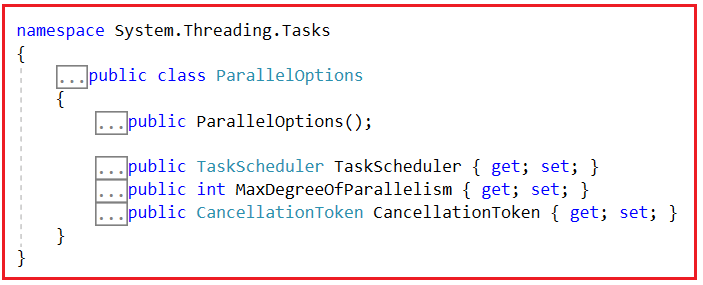The ParallelOptions class in C# provides the following constructor which we can use to create an instance of ParallelOptions class.

The ParallelOptions class provides the following three properties.

1. public TaskScheduler TaskScheduler {get; set;}: It is used to get or set the TaskScheduler associated with this ParallelOptions instance. Setting this property to null indicates that the current scheduler should be used. It returns the task scheduler that is associated with this instance.
2. public int MaxDegreeOfParallelism {get; set;}: It is used to get or set the maximum number of concurrent tasks enabled by this ParallelOptions instance. It returns an integer that represents the maximum degree of parallelism. It will throw ArgumentOutOfRangeException if the property is being set to zero or to a value that is less than -1. -1 is the default value which sets that there is no limitation of the concurrent tasks to be executed.
3. public CancellationToken CancellationToken {get; set;}: It is used to get or set the CancellationToken associated with this ParallelOptions instance. It returns the token that is associated with this instance.

So, in order to use Maximum Degree of Parallelism in C#, we need to create an instance of ParallelOptions class and we need to set the MaxDegreeOfParallelism properties to an integer number indicating the number of threads to execute the code. The Following image shows the syntax to use the Maximum Degree of Parallelism. Here, we set the value to 3 which means a maximum of three threads are going to execute the code parallelly.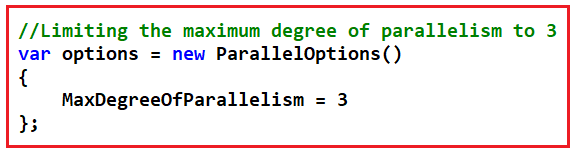Once you create the instance ParallelOptions class and set the MaxDegreeOfParallelism property, then we need to pass this instance to the Parallel methods. The following image shows how to pass the ParallelOptions instance to the Parallel For method in C#.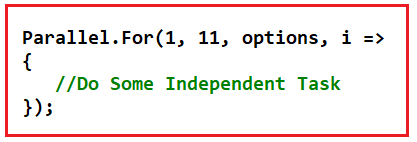With this change, now a maximum of three threads are going to execute the Parallel For loop. The following is the complete code example.

```using System;

namespace ParallelProgrammingDemo
{
class Program
{
static void Main(string[] args)
{
//Limiting the maximum degree of parallelism to 3
var options = new ParallelOptions()
{
MaxDegreeOfParallelism = 3
};

//A maximum of three threads are going to execute the code parallelly
Parallel.For(1, 11, options, i =>
{
});

}
}
}
```
###### Output: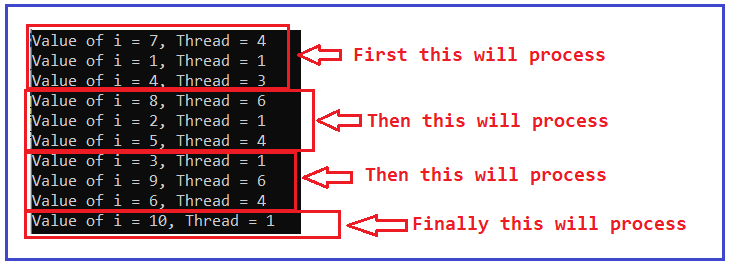When you run the application, please observe the output carefully. First, it will process the first three records, then it will process the next three records, then it will process the next three print statements and finally, it will process the last statement. So, it will not execute all the statements parallelly using different threads rather it will execute the loop using a maximum of three threads parallelly. It might be possible that it will use different threads in each batch.

##### How to properly use the Maximum Degree of Parallelism in C#?

As per the industry standard, we need to set the Maximum Degree of Parallelism to the number of processors available in the machine minus 1. In order to check the number of processors on your machine, open Task Manager and then select the Performance tab and select CPU as shown in the below image.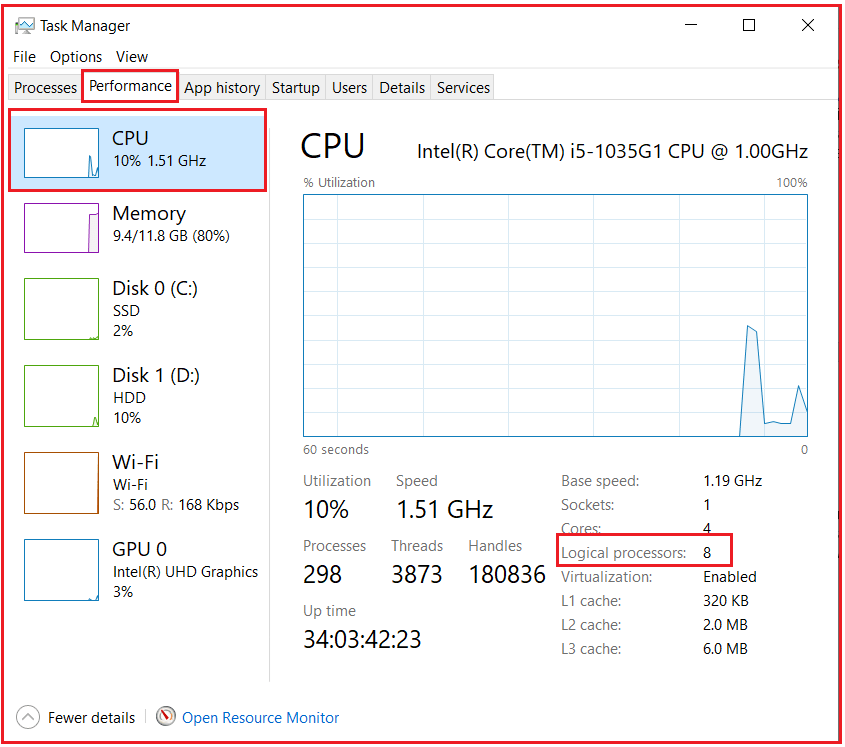As you can see in the above image, on my machine I have 8 Logical Processors. So, as per industry standard, if I want to run the application on my machine then I need to set the Maximum Degree of Parallelism to 7. I can hardcode this value as follows.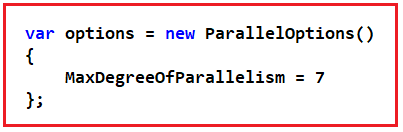But this is not a good programming practice. We are not developing applications to run on our machine. We are developing the application for the client and we don’t know the number of logical processors on the client’s machine. So, we should not hard code the value. Instead, C# provides Environment.ProcessorCount property will give us the number of logical processors on the machine on which the application is running. So, we need to set the Maximum Degree of Parallelism in C# as follows.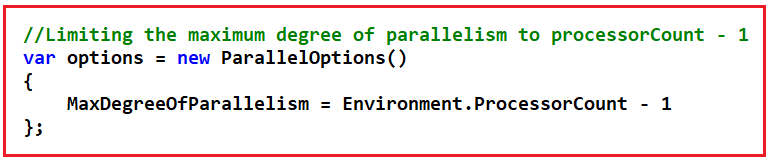The complete example code is given below.

```using System;

namespace ParallelProgrammingDemo
{
class Program
{
static void Main(string[] args)
{
//Getting the Number of Processor count
int processorCount = Environment.ProcessorCount;

Console.WriteLine(\$"Processor Count on this Machine: {processorCount}\n");

//Limiting the maximum degree of parallelism to processorCount - 1
var options = new ParallelOptions()
{
//You can hard code the value as follows
//MaxDegreeOfParallelism = 7
//But better to use the following statement
MaxDegreeOfParallelism = Environment.ProcessorCount - 1
};

Parallel.For(1, 11, options, i =>
{
});

}
}
}
```
###### Output: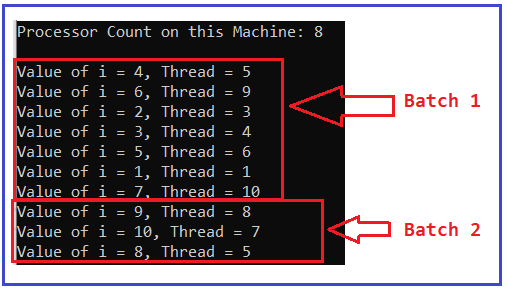I hope now you understand how to effectively use Maximum Degree of Parallelism in C#. Here we have seen the example using the Parallel For loop, the same is applicable to the other two methods i.e. Parallel Invoke and Parallel Foreach loop. Let us see the examples of both.

##### Maximum Degree of Parallelism Example using Parallel Foreach Loop:
```using System;
using System.Collections.Generic;
using System.Linq;

namespace ParallelProgrammingDemo
{
class Program
{
static void Main(string[] args)
{
//Limiting the maximum degree of parallelism to ProcessorCount - 1
var options = new ParallelOptions()
{
//MaxDegreeOfParallelism = 7
MaxDegreeOfParallelism = Environment.ProcessorCount - 1
};

List<int> integerList = Enumerable.Range(0, 10).ToList();
Parallel.ForEach(integerList, options, i =>
{
});

}
}
}
```
##### Maximum Degree of Parallelism Example using Parallel Invoke Method in C#:
```using System;

namespace ParallelProgrammingDemo
{
class Program
{
static void Main(string[] args)
{
var parallelOptions = new ParallelOptions()
{
MaxDegreeOfParallelism = 3
//MaxDegreeOfParallelism = Environment.ProcessorCount - 1
};

//Passing ParallelOptions as the first parameter
Parallel.Invoke(
parallelOptions,
);

}

{
//Sleep for 5000 milliseconds
}
}
}
```
###### Output: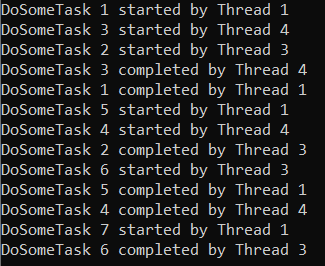In the next article, I am going to discuss How to Cancel Parallel Operations in C# with Examples. Here, in this article, I try to explain How to use the Maximum Degree of Parallelism in C# with Examples. I hope you enjoy this How to use Maximum Degree of Parallelism in C# with Examples.

### 1 thought on “Maximum Degree of Parallelism in C#”

1.Guys,
Please give your valuable feedback. And also, give your suggestions about this Maximum Degree of Parallelism in the C# concept. If you have any better examples, you can also put them in the comment section. If you have any key points related to the Maximum Degree of Parallelism in C#, you can also share the same.